# Bert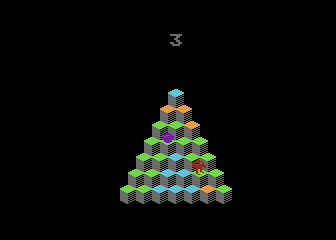This is a little game for the 2022 NOMAM's BASIC 10-liners Contest. This program fits in the PUR-120 category, and it was written in FastBasic 4.5.1 for the 8-bits ATARI XL/XE. Development started on 2020-05-12, and it took 6 days. The final version's date is 2022-03-20.

UPDATE: It obtained the 4th place of 13 entries in the category.

## Description

Bert has to change the color of the 5 pyramids to green, but Bally tries to make him fail the mission.

## InstructionsBert starts in the top of the first pyramid. This is the only safe spot in it. At top appears the number of lives.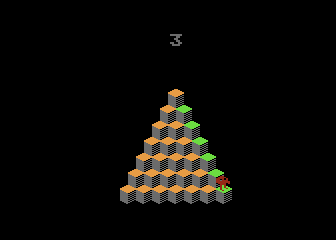Use your joystick to move Bert though the pyramid. The joystick has to be rotated 45° to the right, i.e. you should use it with the red button centered on top.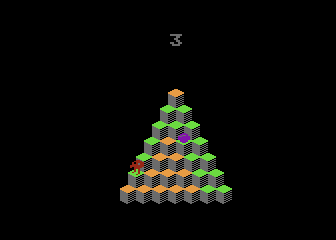From time to time, an enemy called Bally will travel through the pyramid. Avoid him!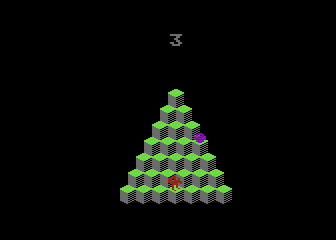Every time you reach a spot, its color might change. Try to change all the spots to green in order to complete the pyramid.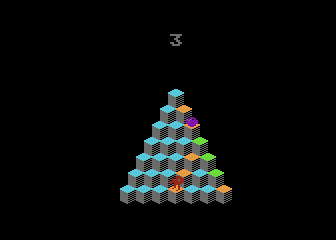While in the first pyramid you can get the green at the first jump in to it, in other pyramids you need to jump more times into every spot in order to make them all green.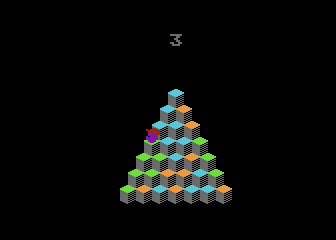Every time Bally gets Bert, a try is lost. Bally will increase his speed and frecuency as time runs.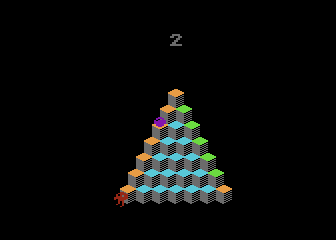If you make Bert jump out of the pyramid, you will also loose a try, and all the work already done in that pyramid will be lost.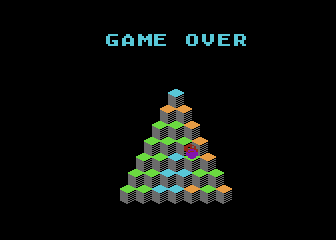When there are no more tries, the mission has failed and the game is over. Press the button to try again.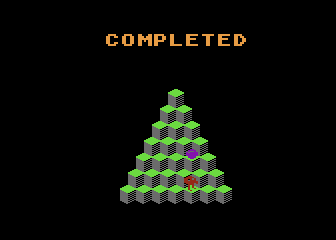You win only when all 5 pyramids were converted to green ones.

## Development of the game

I've always liked the Q*bert character, and decided to program his game for the tenliners contest. I started with a simple pyramid design in graphics mode 12 (ANTIC mode 4) in order to test how it would look and if I could manage all of its states in TurboBASIC XL or FastBasic. I started coding a prototype in FastBasic, because I also designed the sprite for Q*bert and I wanted to see him moving through the pyramid. I also found a formula for the color transitions of the cubes, 5 different sequences for that amount of levels, and it looked great.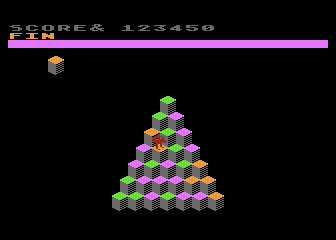Proof of concept

I was surprised that the code to make the character move through the pyramid without smooth transitions between spots was very simple, so I rewote it in Atari BASIC to measure how much space it would require. Of course, I ignored the bitmaps and sprites, and selected the text mode 2 (ANTIC mode 7) to display the pyramid and the character. That branch of this project was called Hubert, and it was submitted to 2021 BASIC tenliner contest for the PUR-80 category.Hubert, a text based version of Q*bert

Back to this project, I realized that the graphics and sprites used too much coding space for the PUR-120 category, the one I was pointing to, so it was archived. Moving to EXTREM-256 was a step I didn't want to do for this game.

More than a year later, being lack of ideas for the 2022 contest, I reviewed my archived projects, trying to find some to finish. With the experience of Hubert, I decided to remove some of the features I planned for the game, in order to fit it in 1200 characters (10 lines of 120 chars each).

I borrowed back the enemy routine from Hubert, but the cost was to remove the random colors for the cubes. The game didn't have sound, so I also got them from Hubert, but I had to do many code optimizations to fit them. By this time, I had decided to cut down the project in order to include some other pending items, so I removed the score and the target cube (there won't be random colors) from the screen. This game became a graphical version of Hubert, with similar gameplay and controls.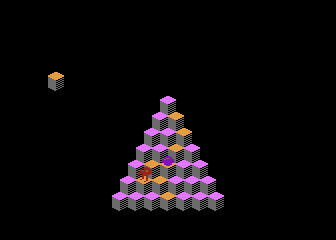Prototype with a moving opponent like in Hubert

My original ideas for this project are still there. If I have enough time, I'll try to include them in a EXTREM-256 version of it.

Get the BERT.ATR file and set it as drive 1 in a real Atari (or emulator). Turn on the computer and the game should start after loading. A joystick in port 1 is required, and it has to be rotated 45° clockwise to play.

## The code

The abbreviated BASIC code is the following: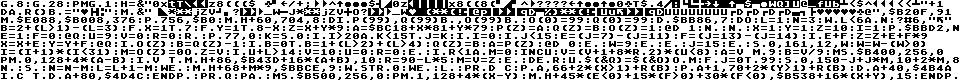The full and expanded BASIC listing is:

 Bert (c) 2020-2022 Víctor Parada 2022-03-20 \$A150-\$BC20 cleaned area by GR.8 \$B000 FastBasic PMBASE when in GR.0 \$BC40 GR.0 screen data area dpeek(88) Not used! \$BBA0 GR.12+16 screen data area dpeek(88) \$BB80........GR.12+16 display list dpeek(560) ```graphics 8 ``` Cleans 8K of top RAM ```graphics 28 ``` Sets 40x24 ANTIC 4 mode ```pmgraphics 1 ``` Enables P/M; graphics, single line resolution ```h=adr("{binary data}")+1 ``` Binary data - P/M bitmaps for Bert (60 bytes) - Color palette (8 bytes) - Completed message (9 bytes) - Game over message (9 bytes) - P/M bitmap for the enemy (10 bytes) ```data r() byte = "{binary data}" ``` Color offsets list ```move adr("{binary data}"),\$B20F,91 ``` CHARSET: New charset is stored at PMBASE (in the unused P/M area) Defines bitmaps for some of the second half for ANTIC 4 mode ```move \$E088,\$B008,376 ``` Copies the first half to be used by scoreboard in ANTIC 7 mode, shifted by 16 chars but without the zero ```poke 756,\$B0 ``` Enables new charset ```move h+60,704,8 ``` - Color palette (9) ```dim p(99),q(99) byte,o(99) byte ``` Cubes arrays - P: Screen position of a cube - Q: Current color of a cube - O: Target color of a cube ```o(0)=99 q(0)=99 ``` Prepares arrays as strings to be compared in a single string expression ```dpoke \$BB86,7 ``` Modifies the Display List: replaces 2 lines with a graphics mode 2 at the TOP, followed by a blank scan line to partially compensate ```do ``` Main loop ``` l=1 ``` Starting level ``` n=3 ``` Lives ``` while l<6 and n ``` Round loop 5 levels while alive ``` print #6,"{binary data}" ``` Clears the playfield ``` b=2+(l>1)-(l=3) ``` Initial color of the cubes ``` for x=1 to 7 for y=1 to 8-x ``` Draws the cubes ``` z=x+y*9 ``` Relative position of the cube ``` a=\$BC18+x*81+y*79 ``` Position in screen of a cube ``` p(z)=a ``` Sets position of the cube ``` q(z)=b ``` Sets color of the cube ``` o(z)=1 ``` Sets cube as valid ``` exec d 1 next y next x ``` Put the cube in the playfield ``` x=1 y=1 z=10 ``` Initial position of the player ``` i=1 ``` Timer ``` poke \$BBD2,n ``` Displays the available lifes ``` e=1 f=0 exec q ``` Displays the player at the initial position ``` u=9 v=0 ``` Enemy position ``` r=0 ``` Enemy delay timer ``` repeat ``` Game loop ``` poke 77,0 ``` Disables ATRACT mode ``` k=stick(0) ``` Delays joystick read ``` if i>20 and k<15 then j=k ``` Saves joystick position if moved in the second half of the wait ``` if i=0 ``` Checks if it's time to move ``` if j<15 ``` Joystick moved? ``` e=(j=7)-(j=11) ``` Moved in X axis? ``` f=(j=13)-(j=14) ``` Moved in Y axis? ``` if e+f ``` Moved? ``` z=z+e+f*9 x=x+e y=y+f ``` Updates current position ``` exec q ``` Displays the player in the new position ``` if o(z) ``` Checks if its stil in the pyramid ``` b=q(z)-1 if b=0 then b=1+(l>2)+(l>4) ``` Calculates new color of the destination cube ``` q(z)=b a=p(z) ``` Assigns new color to the cube ``` exec d 0 endif ``` Displays the new cube ``` w=9 endif endif ``` Enables jump FX ``` j=15 endif ``` Releases joystick (in the buffer) ``` sound 0,161,12,w ``` Jumping FX ``` w=w-(w>0) ``` Next step for the jump FX ``` i=(i+1)*(i<31) ``` ``` m=o(z)=0 or z=v ``` Fell out or hit the enemy? ``` if u+l>14 ``` Triggers the enemy ``` v=10 ``` Puts the enemy in the top ``` u=0 ``` Counter for the number of moves ``` r=0 endif ``` Unsets the delay to activate it ``` if r<1 and m=0 inc u ``` Move enemy? ``` v=(v+1+8*rand(2))*(u<8) ``` Calculates next position ``` a=v mod 9 b=v/9 ``` Computes the new coordinates ``` mset \$B400,256,0 ``` Removes the bitmap ``` pmhpos 0,128+4*(a-b) ``` Moves the player to its horizontal position ``` if v then move h+86, \$B43D+16*(a+b),10 ``` Displays the proper bitmap at the vertical position ``` r=90-l*5 ``` Resets the enemy response timer ``` m=v=z endif ``` Hit the player? ``` dec r ``` Decreases enemy response timer ``` until \$(adr(q))=\$(adr(o)) or m ``` Round ends when pyramid is completed or when killed ``` for j=0 to 99 sound 0,150-j+j*m,10+2*m,8 next j sound ``` End of round FX: wheep for success, brrrr when failed ``` n=n-m ``` Updates the remaning lives ``` l=l+1-m ``` Sets tyhe next level/round ``` wend ``` End of game loop ``` move h+68+m*9,\$BBCE,9 ``` Displays a final message: completed or game over ``` while strig(0) wend ``` Waits for the trigger to play again ```loop ``` End of main loop ```proc d c ``` Draws a cube. Requires the screen position in A and its color in B, C is the parameter, X and Y are the coordinates of the cube (required to verify edges) ``` poke a,\$42+2*(x>1)+r(b) poke a+1,\$46+2*(y>1)+r(b) ``` Top (color) row ``` dpoke a+40,\$4B4A ``` Middle row ``` if c then dpoke a+80,\$4D4C endproc ``` Displays the bottom of the cube only when it is not updating the color ```proc q ``` Moves the player. X and Y are the coordinates of the destination cube, E and F are the moving direction on both axes (just one is not zero) ``` pause ``` Waits for VBLANK ``` mset \$B500,256,0 ``` Removes the bitmap ``` pmhpos 1,128+4*(x-y) ``` Moves the player to its horizontal position ``` move h+45*(e<0)+15*(f>0)+30*(f<0), \$B538+16*(x+y),15 endproc ``` Displays the proper bitmap at the vertical position. Image is selected based on the last moving direction